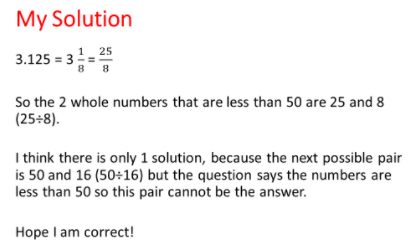#### You may also like### Pebbles

Place four pebbles on the sand in the form of a square. Keep adding as few pebbles as necessary to double the area. How many extra pebbles are added each time?### Bracelets

Investigate the different shaped bracelets you could make from 18 different spherical beads. How do they compare if you use 24 beads?### Sweets in a Box

How many different shaped boxes can you design for 36 sweets in one layer? Can you arrange the sweets so that no sweets of the same colour are next to each other in any direction?

# Forgot the Numbers

##### Age 7 to 11Challenge Level
We had some good solutions submitted for this problem, here is a selection:

Het from Sudbury Primary School  wrote the following:

The two numbers which the person divided by were 25 and 8. I worked this out by using Thomas's work, which said 0.125 is equivalent to 1/8 of 1. The first step I did was to multiply 8 by 0.125 so I got 1. Next I did 3 multiply by 8 to get 24. Next I added 24 and 1 to get 25. I did this because the question says that the sum was a whole divided by a whole so I had to get 3.125 into a whole number. Also we know that whatever the whole you have to divide 1 by 8 to 0.125.
The answer to this question is 25 divided by 8.

Matty from The British School in Manila, Philippines wrote:

The number is 3.125, its last digit is 5 (in the thousandths). Therefore, since the number is a whole number, and it ends in 5, we can keep multiplying by 2 to find the answer.

3.125 * 2 = 6.25
3.125 * 4 = 12.5
3.125 * 8 = 25

We have both numbers, 25 and 8! It cannot be simplified in any way, so our answer is 25 and 8!

A number of pupils from St. Mary's School contributed, namely, Cadence and Joanna; Kaya, Maisie and Arthur.  Here is what they said:

We found out that 0.125 is equal to an eighth, and we knew that 8 multiplied by 3 equals 24,and 8 x 0.125 is 1, and 1 plus 24 is 25, so therefore 25 divided by eight is 3.125.
Thank you for this puzzle.

Tommy Farmer from St Georges Primary in Bickley wrote:

I knew as it ended in 0.125 that it had to be something divided by 8.  So I calculated the inverse, ie, 3.125 x 8 and it gives an answer of 25.
So 25 divided by 8 (both numbers under 50) = 3.125.

Edward from Weston Favell Primary School wrote :

3.125 is equal to 3+1/8.
If we use algebra we can use M and C as the numbers we are looking for.
3 + 1/8 x M = C
I thought that we could times 3 by 8 and 1/8 by 8.  It would equal 24 + 1= 25 so the numbers  would be M = 8, C = 25

Ahan Singhal from Tanglin Trust School, Singapore sent in the following:

Since 0.125 is equal to 1/8, I need to multiply the number by 8 or a multiple of 8 to get a whole number. So, I start with multiplying 3.125 by 8, which gives me 25.
So, one option is 8 and 25.
If I double 25, it gives me 50 which is already outside the biggest number allowed (<50).
Therefore, the only possible answer is 25 and 8.

Tan Sze Qi from Innova Primary School in Singapore sent in the solution as an image.Well done, these showed some good thinking as well as excellent explanations.